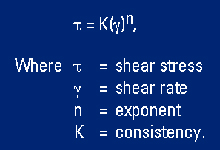# Explore the Energy Glossary

Look up terms beginning with:

# power-law fluid

1. n. []

A fluid described by the two-parameter rheological model of a pseudoplastic fluid, or a fluid whose viscosity decreases as shear rate increases. Water-base polymer muds, especially those made with XC polymer, fit the power-law mathematical equation better than the Bingham plastic or any other two-parameter model. Power-law fluids can be described mathematically as follows: